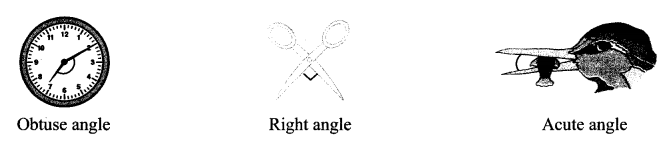Maharashtra Board Class 6 Maths Solutions Chapter 2 Angles Practice Set 2

Maharashtra State Board Class 6 Maths Solutions Chapter 2 Angles Practice Set 2

Question 1.
Match the following:

 Measure of the angle Type of the angle i. 180° a. Zero angle ii. 240° b. Straight angle iii. 360° c. Reflex angle iv. 0° d. Complete angle

Solution:
(i – Straight Angle),
(ii – Reflex Angle),
(iii – Complete Angle),
(iv – Zero Angle).

Question 2.
The measures of some angles are given below. Write the type of each angle:

1. 75°
2. 215°
3. 360°
4. 180°
5. 120°
6. 148°
7. 90°

Solution:

1. Acute angle
2. Zero angle
3. Reflex angle
4. Complete angle
5. Straight angle
6. Obtuse angle
7. Obtuse angle
8. Right angle

Question 3.
Look at the figures below and write the type of each of the angles:Solution:
a. Acute angle
b. Right angle
c. Reflex angle
d. Straight angle
e. Zero angle
f. Complete angle

Question 4.
Use a protractor to draw an acute angle, a right angle and an obtuse angle:
Solution:[Note: Students may draw acute and obtuse angles of measure other than the ones given.]

Maharashtra Board Class 6 Maths Chapter 2 Angles Practice Set 2 Intext Questions and Activities

Question 1.
Look at the angles shown in the pictures below. Identify the type of angle and write its name below the picture: (Textbook pg. no. 6)Solution:Question 2.
Complete the following table: (Textbook pg. no. 6)Solution:

 Sr. No. i. ii. iii. Name of the angle ∠PYR or ∠RYP ∠LMN or ∠NML ∠BOS or ∠SOB Vertex of the angle Y M O Arms of the angle YP and YR ML and MN OB and OS